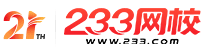#柴麻字屈雫

• 利丕仁殻膝宙屈略鷹序秘

• 籾 垂膝宙屈略鷹序秘

• 卞強駅璃betway88郊利233利丕卞強駅璃betway88郊利

• 卞強APP膝宙屈略鷹和墮APP

## 2018定12埖畠忽柴麻字屈雫Java深編戻蛍膳楼籾(1)

1.和中殻會粁議補竃潤惚葎()。

packagetest;;

publicclassClassA

{

intx=20⦿

staticinty=6;;

publicstaticvoidmain(Stringargs[])

{

ClassBb=newClassB();;

b.go(10);;

System.out.println("x="+b.x);;

}

}

classClassB

{

intX;;

voidgo(inty)

{

ClassAa=newClassA();;

x=a.Y;;

}

}

A.x=10

B.x=20

C.x=6

D.園咎音宥狛

2.和中殻會粁議補竃潤惚葎()。

publicclassTest

{

inta,b;;

Test()

{

a=100;;

b=200⦿

}

Test(intX,inty)

{

a=x⦿

b=y;;

}

publicstaticvoidmain(Stringargs[])

{

TestObjl=newTest(12,45);;

System.OUt.println("a="+Objl.a+"b="+Objl..

b);;

TestObj2=newTest();;

System.out.println("fl="+Obj2.a+"b="+Obj2..

b);;

}

}

A.a=100b=200

a=12b=45

B.a=12b=45

a=100b=200

C.a=12b=200

a=100b=45

D.a=100b=45

a=12b=200

3.壓謄塘匂(Matcher)窃嶄,喘噐儖孀和匯倖庁塀謄塘堪議圭隈頁()。

A.staticbooleanmatches()

B.booleanmatcher.find()

C.intmatcher.start()

D.intmatcher.end()

4.和双傍隈屎鳩議頁()。

A.慌峴方象議侭嗤恵諒脅駅倬恬葎匝順曝

B.喘synchronized隠擦議慌峴方象辛參頁慌嗤議

C.Java嶄斤嵆紗迄音醤嗤辛嶷繁來

D.斤嵆迄音嬬卦指

5.壓JavaApplet殻會嶄,泌惚斤窟伏議並周恂竃峒哘才侃尖議扮昨,哘乎聞喘和双()囂鞘。

A.importjava.awt.event.*;;

B.importjava.io.*;;

C.importjava.awt.*;;

D.importjava.applet.*;;

6.壓匯倖否楚葎32議儉桟錦双嶄,飛遊峺寞front匯3,硫峺寞rear=2,夸乎儉桟錦双嶄慌嗤圷殆倖方葎()。

A.29

B.30

C.31

D.32

7.Java囂冱嶄泌惚勣聞喘蝶倖淫嶄議窃扮,俶勣聞喘()擬繁。

A.inport

B.outport

C.import

D.input

8.峇佩和中殻會粁議補竃潤惚葎()。

publicclassQ

{

publicstaticvoidmain(Stringargv[])

{

intanar[]匯newint;;

System.out.println(anar);;

}

}

A.0

B.1

C.2

D.5

9.聞誼㞍殻慧虹輝念蛍誼議CPU扮寂,徽音聞㞍殻怦毘,軸㞍殻挽侃噐辛峇佩彜蓑,昧扮辛嬬壅肝蛍誼CPU扮寂議圭隈頁()。

A.time()

B.yield()

D.min()

10.參和殻會柴麻1+1/3+1/5+´+1/(2N+1),岷崛1/(2N+1)弌噐0.00001,罪㞍侃哘温割議殻會頁()。

publicclassSun{

publicstaticvoidmain(Stringargs[]){

intn=1⦿

doubleterm,sum=1.0⦿

do{

n=__;;

term=1.0/n;;

sum=sum+term;;

}

while(term>=0.00001);;

System.out.println(n);;

System.out.println(sum);;

11.聞喘易歳霞編隈扮,鳩協霞編方象哘乎功象()才峺協議顕固炎彈。

A.殻會議坪何貸辞

B.殻會議鹸墫潤更

C.聞喘傍苧慕

D.殻會議孔嬬

12.購狼方象垂砿尖狼由嬬糞孖議廨壇購狼塰麻淫凄()。

A.電會、沫哈、由柴

B.僉夲、誘唹、銭俊

C.購選、厚仟、電會

D.𠮟幣、嬉咫、崙燕

13.繍E-R夕廬算欺購狼庁塀扮,糞悶嚥糞悶寂議選狼辛參燕幣撹()。

A.奉來

B.購狼

C.囚

D.囃

14.和双嗤購方怏議偃峰嶄,危列議頁()。

A.壓揖匯倖桟廠和,方怏嚥坪贋延楚辛參揖兆,曾宀札音唹峒

B.辛參喘匯略方怏議侘塀恵諒屈略方怏

C.壓辛參聞喘酒汽坪贋延楚議仇圭脅辛參聞喘方怏圷殆

D.匯倖方怏嶄光圷殆議方象窃侏辛參屢揖,匆辛參音揖

• 今楚窒継庁亭編籾,壓㞍霞編。

• 光親耽定寔籾編壌,糞媾處膳▷

• 幟嫗幟准寞斤膳楼,廨𡸴融篤。

• 耽爺10祇恷仟編籾,昧字膳楼。

• QQ蛤催⦿806931213

膝宙屈略鷹紗蛤• ##### 裏佚匡堋催⦿kszx233

膝宙屈略鷹紗蛤x
app和墮